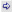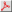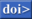BACK TO INDEX BACK TO OTHMAR FREY'S HOMEPAGE

## Publications of year 1972

### Articles in journal or book chapters

1. J. Saastamoinen. Atmospheric Correction for the Troposphere and Stratosphere in Radio Ranging Satellites, pages 247-251. American Geophysical Union, 1972.Keyword(s): Meteorology, Radio ranging of satellites, Refractive index of air, Stratosphere, Troposphere.
 Abstract: Since the barometer measures the weight of the overlying atmosphere, it follows by the law of Gladstone and Dale that the height integral int (n - 1)dr of the atmospheric refractivity for radio microwaves, taken from ground level up to the top of the stratosphere, is in a dry atmosphere directly proportional to ground pressure. The refractivity integral, therefore, can be determined without detailed knowledge of the height distribution of the refractive index, which not only simplifies the derivation of refraction formulas in which atmospheric models have been used hitherto, but also improves their accuracy. The range correction for troposphere and stratosphere to be subtracted from the observed microwave distance will be given by the formula ds (meters) = 0.002277 sec z [p + (1255/T + 0.05)e - 1.16 tan^2 z] where z is the zenith distance, p is the total barometric pressure and e is the partial pressure of water vapor, both in millibars, and T is the absolute temperature in degrees Kelvin.

@InBook{saastamoinen1972AtmosphericPathDelay,
pages = {247--251},
title = {Atmospheric Correction for the Troposphere and Stratosphere in Radio Ranging Satellites},
publisher = {American Geophysical Union},
year = {1972},
author = {Saastamoinen, J.},
editor = {S. W. Henriksen and A. Mancini and B. H. Chovitz},
isbn = {9781118663646},
abstract = {Since the barometer measures the weight of the overlying atmosphere, it follows by the law of Gladstone and Dale that the height integral int (n - 1)dr of the atmospheric refractivity for radio microwaves, taken from ground level up to the top of the stratosphere, is in a dry atmosphere directly proportional to ground pressure. The refractivity integral, therefore, can be determined without detailed knowledge of the height distribution of the refractive index, which not only simplifies the derivation of refraction formulas in which atmospheric models have been used hitherto, but also improves their accuracy. The range correction for troposphere and stratosphere to be subtracted from the observed microwave distance will be given by the formula ds (meters) = 0.002277 sec z [p + (1255/T + 0.05)e - 1.16 tan^2 z] where z is the zenith distance, p is the total barometric pressure and e is the partial pressure of water vapor, both in millibars, and T is the absolute temperature in degrees Kelvin.},
booktitle = {The Use of Artificial Satellites for Geodesy},
doi = {10.1029/GM015p0247},
file = {:saastamoinen1972AtmosphericPathDelay.pdf:PDF},
keywords = {Meteorology, Radio ranging of satellites, Refractive index of air, Stratosphere, Troposphere},
pdf = {../../../docs/saastamoinen1972AtmosphericPathDelay.pdf},
url = {http://dx.doi.org/10.1029/GM015p0247},

}


2. J. Saastamoinen. Contributions to the theory of atmospheric refraction. Bulletin Géodésique (1946-1975), 105(1):279-298, 1972.Abstract: Since the barometer measures the weight of the overlying atmosphere, it follows by the law of Gladstone and Dale that the height integral of the atmospheric refractivity for light, taken from ground level up to the top of the atmosphere, is directly proportional to ground pressure. The refractivity integral, therefore, can be determined without detailed knowledge of the height distribution of the refractive index, which not only simplifies the derivation of refraction formulas in which atmospheric models have been used hitherto, but also improves their accuracy. For zenith distances not exceeding about 75 degrees, the correction for astronomical refraction will be given by the standard formula$$\begin{gathered} \Delta z''_0 = 16''.271 tan z\left[ {1 + 0.0000394 tan^2 z\left( {\frac{{p - 0.156e}}{T}} \right)} \right]\left( {\frac{{p - 0.156e}}{T}} \right) - \hfill \\ - 0''.0749 (tan^3 z + tan z)\left( {\frac{p}{{1000}}} \right) \hfill \\ \end{gathered}$$wherez is the apparent zenith distance,p is the total pressure ande is the partial pressure of water vapour, both in millibars, andT is the absolute temperature in degrees Kelvin Part II of the paper contains further applications of the theory to refraction problems in satellite geodesy, including the photogrammetric refraction and the atmospheric corrections in the ranging of artificial satellites.

@Article{saastamoinen1972AtmosphericRefractionPart1,
author = {Saastamoinen, J.},
journal = {Bulletin G\'eod\'esique (1946-1975)},
title = {Contributions to the theory of atmospheric refraction},
year = {1972},
issn = {0007-4632},
number = {1},
pages = {279--298},
volume = {105},
abstract = {Since the barometer measures the weight of the overlying atmosphere, it follows by the law of Gladstone and Dale that the height integral of the atmospheric refractivity for light, taken from ground level up to the top of the atmosphere, is directly proportional to ground pressure. The refractivity integral, therefore, can be determined without detailed knowledge of the height distribution of the refractive index, which not only simplifies the derivation of refraction formulas in which atmospheric models have been used hitherto, but also improves their accuracy. For zenith distances not exceeding about 75 degrees, the correction for astronomical refraction will be given by the standard formula$$\begin{gathered} \Delta z''_0 = 16''.271 tan z\left[ {1 + 0.0000394 tan^2 z\left( {\frac{{p - 0.156e}}{T}} \right)} \right]\left( {\frac{{p - 0.156e}}{T}} \right) - \hfill \\ - 0''.0749 (tan^3 z + tan z)\left( {\frac{p}{{1000}}} \right) \hfill \\ \end{gathered}$$wherez is the apparent zenith distance,p is the total pressure ande is the partial pressure of water vapour, both in millibars, andT is the absolute temperature in degrees Kelvin Part II of the paper contains further applications of the theory to refraction problems in satellite geodesy, including the photogrammetric refraction and the atmospheric corrections in the ranging of artificial satellites.},
doi = {10.1007/BF02521844},
file = {:saastamoinen1972AtmosphericRefractionPart1.pdf:PDF},
owner = {ofrey},
refid = {Saastamoinen1972},
url = {https://doi.org/10.1007/BF02521844},

}


BACK TO INDEX BACK TO OTHMAR FREY'S HOMEPAGE

Disclaimer:

Please note that access to full text PDF versions of papers is restricted to the Chair of Earth Observation and Remote Sensing, Institute of Environmental Engineering, ETH Zurich.
Copyright and all rights therein are retained by authors or by other copyright holders. All persons copying this information are expected to adhere to the terms and constraints invoked by each author's copyright.

This collection of SAR literature is far from being complete.
It is rather a collection of papers which I store in my literature data base. Hence, the list of publications under PUBLICATIONS OF AUTHOR'S NAME should NOT be mistaken for a complete bibliography of that author.